Courses

# Test: Dynamic of Fluid Flow - 3

## 10 Questions MCQ Test Topicwise Question Bank for Mechanical Engineering | Test: Dynamic of Fluid Flow - 3

Description
This mock test of Test: Dynamic of Fluid Flow - 3 for Mechanical Engineering helps you for every Mechanical Engineering entrance exam. This contains 10 Multiple Choice Questions for Mechanical Engineering Test: Dynamic of Fluid Flow - 3 (mcq) to study with solutions a complete question bank. The solved questions answers in this Test: Dynamic of Fluid Flow - 3 quiz give you a good mix of easy questions and tough questions. Mechanical Engineering students definitely take this Test: Dynamic of Fluid Flow - 3 exercise for a better result in the exam. You can find other Test: Dynamic of Fluid Flow - 3 extra questions, long questions & short questions for Mechanical Engineering on EduRev as well by searching above.
QUESTION: 1

### The Bernoulli’s equation refers to conservation of

Solution:

Bernoulli’s equation refers to conservation of energy.

QUESTION: 2

### How are the velocity coefficient Cv, the discharge coefficient Cd and the contraction coefficient Cc of an orifice related

Solution:

The relation between Cd, Cc and Cv is
Cd = CcCv

QUESTION: 3

### The coefficient of velocity (Cv) for an orifice is

Solution: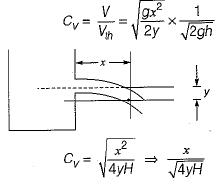QUESTION: 4

The equation of motion for a viscous fluid known as

Solution:

In Navier-Stokes equation pressure force, gravity force and viscous force are considered.

QUESTION: 5

The rate of flow through venturimeter varies as

Solution:

Rate of flow through a venturimeter is given as: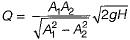Hence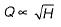QUESTION: 6

A smooth pipe of diameter 200 mm carries water. The pressure in the pipe at section S1 (elevation:10 m) is 50 kPa. At section S2 (elevation:12 m) the pressure is 20 kPa and velocity is 2 ms-1. Density of water is 1000 kgm-3 and acceleration due to gravity is 9.8 ms-2. Which of the following is TRUE

Solution: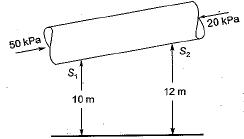Applying Bernoulli’s equation between section S1 and S2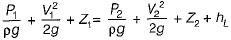as area is constant velocity head will be same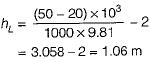thus flow from S1 to S2 and head loss is 1.06 m.

QUESTION: 7

While measuring the velocity of air ρ = 1.2 kg/m3, the difference in the stagnation and static pressure of a Pitot-static tube was found to be 380 Pa. The velocity at that location in m/s is

Solution: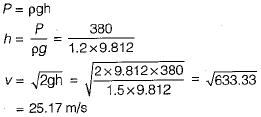QUESTION: 8

If a fluid jet discharging from a 50 mm diameter orifice has a 40 mm diameter at its vena contracta, then its coefficient of contraction will be

Solution: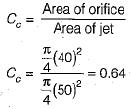QUESTION: 9

Match List-I with List-il and select the correct answer using the codes given below the lists:
List-I
A. Orifice meter
C. Pitot tube
D. Rotameter
List-Il
1. Measurement of flow in a channel
2. Measurement of velocity in a pipe/channel
3. Measurement of flow in a pipe of any inclination
4. Measurement of upward flow in a vertical pipe
Codes:
A B C D
(a) 3 1 4  2
(b) 1 3 2  4
(c) 3 1 2  4
(d) 1 3 4  2

Solution:
QUESTION: 10

A Pitot tube is used to measure the velocity of water in a pipe. The stagnation pressure head is 6 m and the static pressure head is 5 m. If the coefficient of tube is 0.98, then what is the velocity of flow?

Solution: